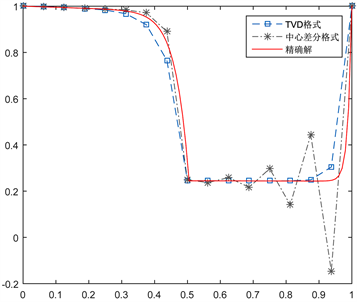# Fokker-Planck方程TVD有限体积方法TVD Finite Volume Method for Fokker-Planck Equation

DOI: 10.12677/AAM.2021.104133, PDF, HTML, XML, 下载: 8  浏览: 23

Abstract: We study a TVD finite volume method for solving the time fractional Fokker-Planck equation, in which the TVD scheme and the central difference scheme are used to discretize the convection term and the diffusion term respectively, and L1 scheme is used to discretize the time fractional derivative. The numerical tests show that our method has advantages when it is used to solve convection dominated problems on coarse grids.

1. 研究问题

$\frac{{\partial }^{\alpha }w}{\partial {t}^{\alpha }}=\left({k}_{\alpha }\frac{{\partial }^{2}}{\partial {x}^{2}}-\frac{\partial }{\partial x}f\left(x\right)\right)w\left(x,t\right),a\le x\le b,0\le t\le T,$ (1.1)

$w\left(x,0\right)=\phi \left(x\right),a\le x\le b,w\left(a,t\right)={g}_{1}\left(t\right),w\left(b,t\right)={g}_{2}\left(t\right),0\le t\le T,$ (1.2)

$\alpha \left(0<\alpha <1\right)$ 阶Caputo分数阶导数： $\frac{{\partial }^{\alpha }w}{\partial {t}^{\alpha }}=\frac{1}{\text{Γ}\left(1-\alpha \right)}\frac{\partial }{\partial t}{\int }_{0}^{t}\frac{\partial w\left(x,s\right)}{\partial t}\frac{\text{d}s}{{\left(t-s\right)}^{\alpha }}$$\Gamma \left(\alpha \right)$ 是Gamma函数。方程(1.1)

${\int }_{{x}_{i-\frac{1}{2}}}^{{x}_{i+\frac{1}{2}}}{\frac{{\partial }^{\alpha }w}{\partial {t}^{\alpha }}|}_{{t}_{n}}={k}_{\alpha }\left[{\left(\frac{\partial w}{\partial x}\right)}_{i+\frac{1}{2}}^{n}-{\left(\frac{\partial w}{\partial x}\right)}_{i-\frac{1}{2}}^{n}\right]-\left[{\left(fw\right)}_{i+\frac{1}{2}}^{n}-{\left(fw\right)}_{i-\frac{1}{2}}^{n}\right],i=1,2,\cdots ,N.$ (1.3)

$\begin{array}{l}\frac{h\Delta {t}^{-\alpha }}{\Gamma \left(2-\alpha \right)}\left({w}_{i}^{n}-\underset{k=1}{\overset{n-1}{\sum }}\left({a}_{n-k-1}-{a}_{n-k}\right){w}_{i}^{k}-{a}_{n-1}{w}_{i}^{0}\right)\\ =\frac{{k}_{\alpha }}{h}\left({w}_{i+1}^{n}-2{w}_{i}^{n}+{w}_{i-1}^{n}\right)-{\left(fw\right)}_{i+\frac{1}{2}}^{n}+{\left(fw\right)}_{i-\frac{1}{2}}^{n},\text{\hspace{0.17em}}\text{\hspace{0.17em}}i=1,2,\cdots ,N\end{array}$ (1.4)

2. TVD格式离散对流项

${\left(fw\right)}_{i+\frac{1}{2}}^{n}={f}_{i+\frac{1}{2}}\left[{w}_{i}^{n}+\frac{1}{2}\psi \left({r}_{i+\frac{1}{2}}^{+}\right)\left({w}_{i+1}^{n}-{w}_{i}^{n}\right)\right],{f}_{i+\frac{1}{2}}>0$

${\left(fw\right)}_{i+\frac{1}{2}}^{n}={f}_{i+\frac{1}{2}}\left[{w}_{i+1}^{n}-\frac{1}{2}\psi \left({r}_{i+\frac{1}{2}}^{-}\right)\left({w}_{i+1}^{n}-{w}_{i}^{n}\right)\right],{f}_{i+\frac{1}{2}}<0.$ (2.1)

${r}_{i+\frac{1}{2}}^{+}=\frac{{w}_{i}^{n}-{w}_{i-1}^{n}}{{w}_{i+1}^{n}-{w}_{i}^{n}},{r}_{i+\frac{1}{2}}^{-}=\frac{{w}_{i+2}^{n}-{w}_{i+1}^{n}}{{w}_{i+1}^{n}-{w}_{i}^{n}}.$ (2.2)

${F}_{i}\left({w}_{i-1}^{n},{w}_{i}^{n},{w}_{i+1}^{n}\right)+{G}_{i}\left({w}_{i-1}^{n},{w}_{i}^{n},{w}_{i+1}^{n}\right)=\frac{h\Delta {t}^{-\alpha }}{\Gamma \left(2-\alpha \right)}\left({\sum }_{k=1}^{n-1}\left({a}_{n-k-1}-{a}_{n-k}\right){w}_{i}^{k}-{a}_{n-1}{w}_{i}^{0}\right).$ (2.3)

$\begin{array}{c}{F}_{i}\left({w}_{i-1}^{n},{w}_{i}^{n},{w}_{i+1}^{n}\right)=\frac{1}{2}{f}_{i+\frac{1}{2}}\left[\beta \psi \left({r}_{i+\frac{1}{2}}^{+}\right)-\stackrel{¯}{\beta }\psi \left({r}_{i+\frac{1}{2}}^{-}\right)\right]\left({w}_{i+1}^{n}-{w}_{i}^{n}\right)\\ \text{\hspace{0.17em}}-\frac{1}{2}{f}_{i-\frac{1}{2}}\left[\beta \psi \left({r}_{i-\frac{1}{2}}^{+}\right)-\stackrel{¯}{\beta }\psi \left({r}_{i-\frac{1}{2}}^{-}\right)\right]\left({w}_{i}^{n}-{w}_{i-1}^{n}\right)\end{array}$ (2.4)

$\begin{array}{c}{G}_{i}\left({w}_{i-1}^{n},{w}_{i}^{n},{w}_{i+1}^{n}\right)=-\left[\mathrm{max}\left({f}_{i-\frac{1}{2}},0\right)+\frac{{k}_{\alpha }}{h}\right]{w}_{i-1}^{n}-\left[\mathrm{max}\left(-{f}_{i+\frac{1}{2}},0\right)+\frac{{k}_{\alpha }}{h}\right]{w}_{i+1}^{n}\\ \text{\hspace{0.17em}}+\left[\mathrm{max}\left({f}_{i-\frac{1}{2}},0\right)+\mathrm{max}\left(-{f}_{i+\frac{1}{2}},0\right)+\left({f}_{i+\frac{1}{2}}-{f}_{i-\frac{1}{2}}\right)+\frac{2{k}_{\alpha }}{h}+\frac{h\Delta {t}^{-\alpha }}{\Gamma \left(2-\alpha \right)}\right]{w}_{i}^{n}\end{array}$ (2.5)

3. 数值实验及结论

$\frac{{\partial }^{\alpha }w}{\partial {t}^{\alpha }}=\left({k}_{\alpha }\frac{{\partial }^{2}}{\partial {x}^{2}}-\frac{\partial }{\partial x}f\left(x\right)\right)w\left(x,t\right)+G\left(x,t\right),0\le x\le 1,0\le t\le 1,$

$w\left(x,0\right)=0,{g}_{1}\left(t\right)={t}^{2}\mathrm{cos}\left(2\pi \right),{g}_{2}\left(t\right)={t}^{2}\mathrm{cos}\left(-2\pi \right).$Figure 1. Comparison of solving effects of N = 15 (left) and N = 25 (right)

  Sokolov, I.M., Blumen, A. and Klafter, J. (2001) Linear Response in Complex Systems: CTRW and the Fractional Fokker-Planck Equations. Physica A: Statistical Mechanics and its Applications, 302, 268-278. https://doi.org/10.1016/S0378-4371(01)00470-8  安德森. 计算流体力学基础及其应用[M]. 北京: 机械工业出版社, 2009.  Fairweather, G., Zhang, H., Yang, X., et al. (2015) A Backward Euler Orthogonal Spline Collocation Method for the time-Fractional Fokker-Planck Equation. Numerical Methods for Partial Differential Equations, 31, 1534-1550. https://doi.org/10.1002/num.21958  Versteeg, H.K. and Malalasekera, W. (2007) Computational Fluid Dynamics: The Finite Volume Method. Pearson Schweiz AG, 20, 400.  van der Vorst, H.A. (1992) Bi-CGSTAB: A Fast and Smoothly Converging Variant of Bi-CG for the Solution of Nonsymmetric Linear Systems. SIAM Journal on Scientific & Statistical Computing, 13, 631-644. https://doi.org/10.1137/0913035